Set 2 (10 Questions)
Add and subtract simple fractions (e.g., determine that 1/8+3/8 is the same as 1/2).
From Mr. Anker Tests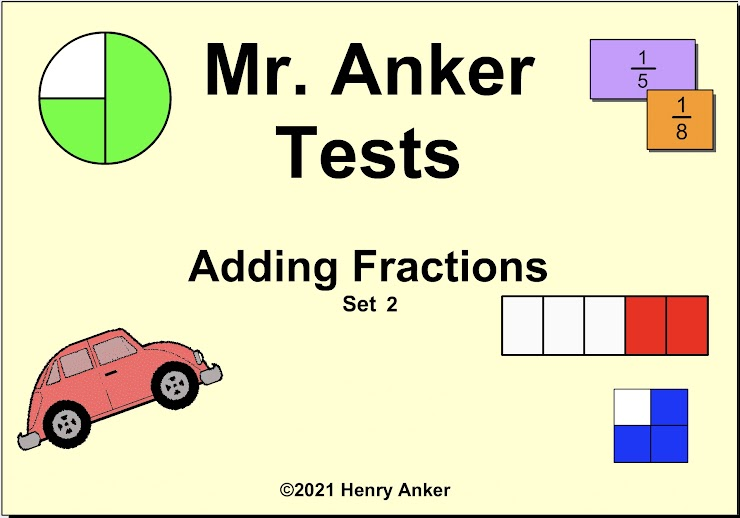1.  What is the sum of 3/8 + 1/8? *
1 point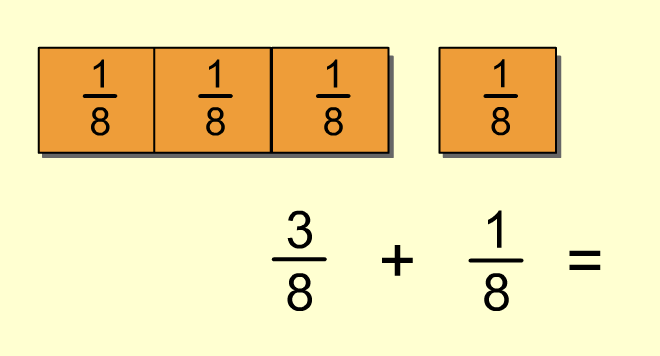2.  What is the sum of 1/3 + 2/3? *
1 point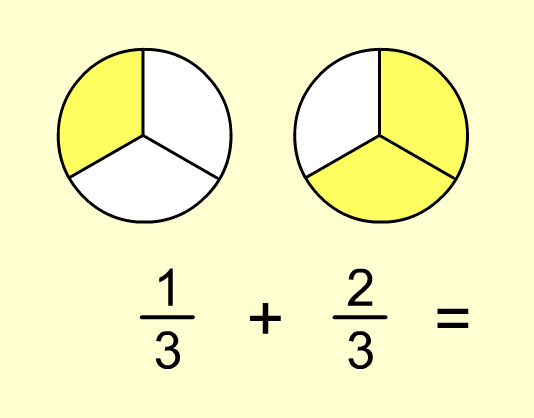3.  What is the sum of 2/4 + 0/4? *
1 point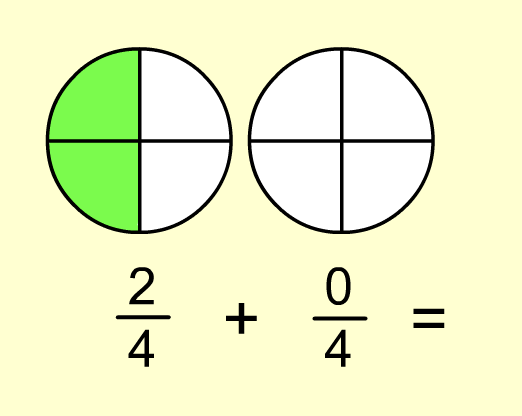4.  What is the sum of 1/6 + 1/6? *
1 point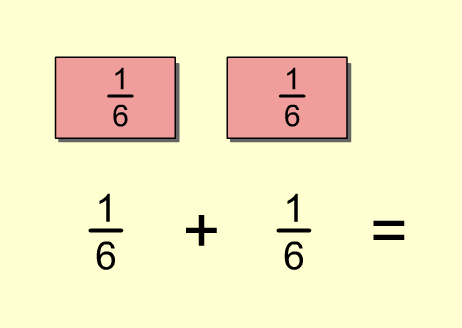5.  What is the sum of 4/16 + 9/16? *
1 point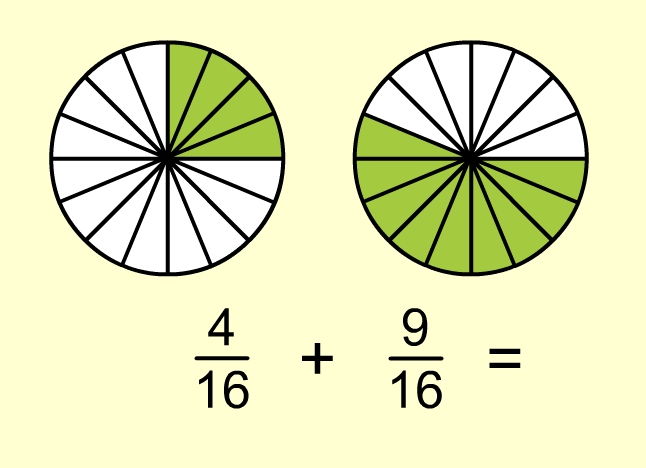6.  What is the sum of 5/6 + 1/6? *
1 point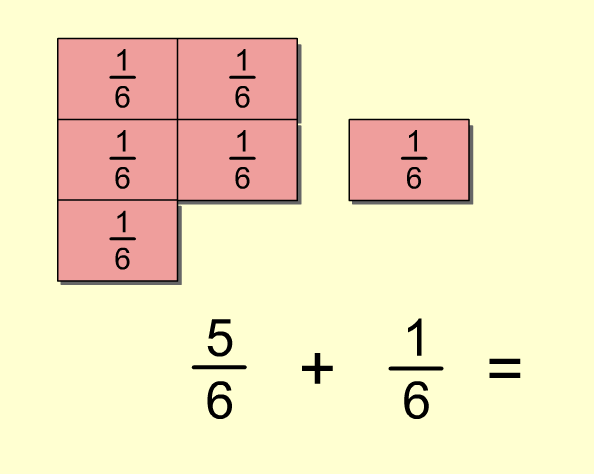7.  What is the sum of 1/6+ 0/6 ? *
1 point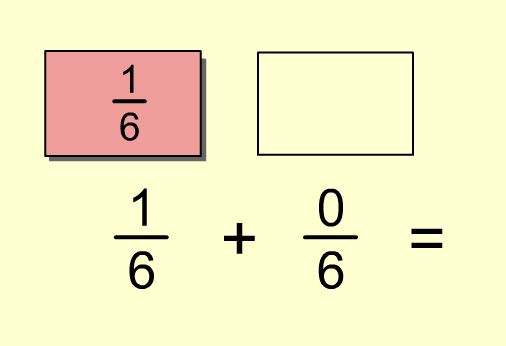8. What is the sum of 3/4+ 2/4? *
1 point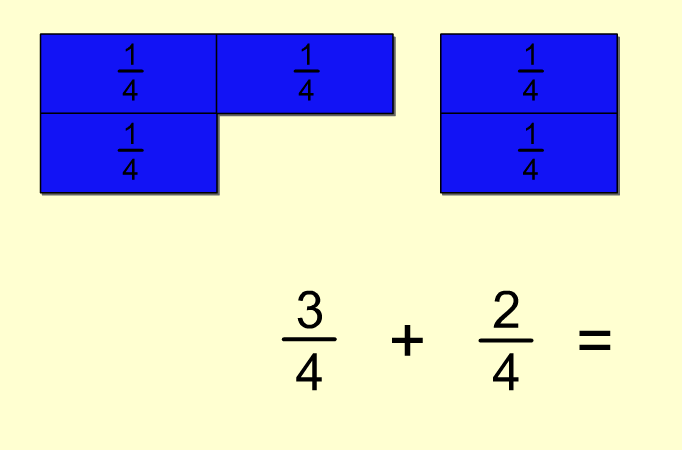9. What is the sum of 5/9+ 6/9? *
1 point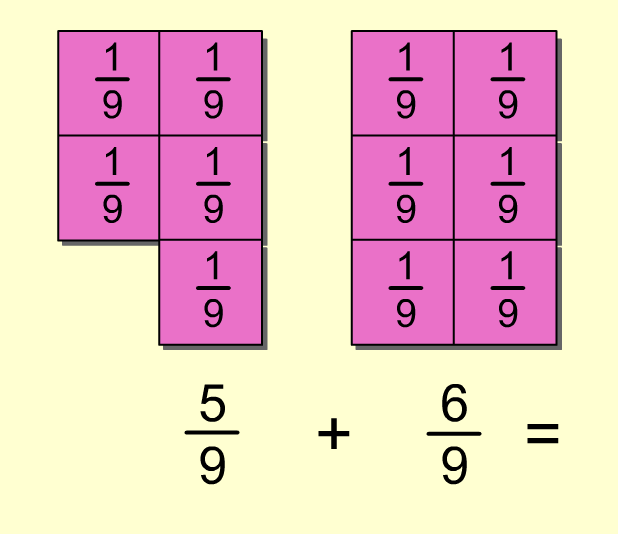10. What is the sum of 3/8+ 1/2? *
1 point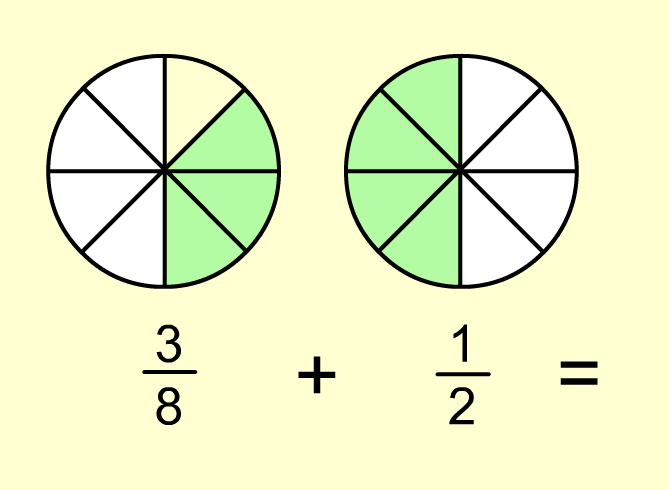Submit
Clear form